# Test: Sequential Circuits- 2

## 15 Questions MCQ Test Question Bank for GATE Computer Science Engineering | Test: Sequential Circuits- 2

Description
Attempt Test: Sequential Circuits- 2 | 15 questions in 45 minutes | Mock test for Computer Science Engineering (CSE) preparation | Free important questions MCQ to study Question Bank for GATE Computer Science Engineering for Computer Science Engineering (CSE) Exam | Download free PDF with solutions
QUESTION: 1

###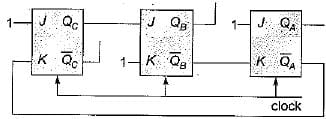If the initial state QA QB QC = 110, after how many clocks it get back same value

Solution: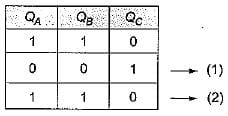QUESTION: 2

###What will be the current state after two clock pulses further from the number of pulses obtained from the above question?

Solution: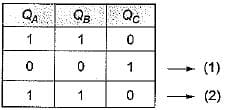QUESTION: 3

### The divide by N counter as shown in figure. If initially Q0 = 0, Q1 = 1, Q2 = 0. What is the value of N?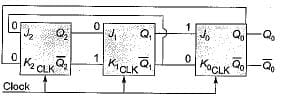Solution: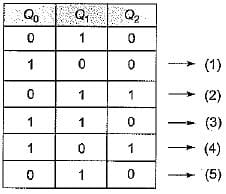So 5-mod counter.

QUESTION: 4

​Consider the circuit given below with initial state Q0 = 1; Q1 = Q2 = 0. The state of the circuit is given by the value 4Q2 + 2Q1 + Q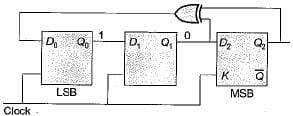Which one of the following is the. correct state sequence of the circuit?

Solution: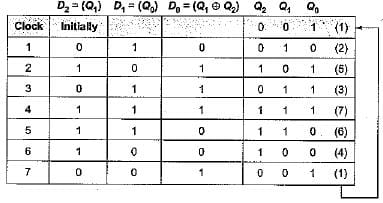Therefore, the correct state sequence of the circuit is 1, 2, 5, 3, 7, 6, 4.

QUESTION: 5

The following diagram represents a finite state machine which takes as input a binary number from the least significant bit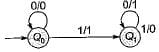Which one of the following is TRUE?

Solution: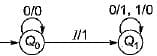Here, for state Q0
When input is 0 output is 0 and it remains in state Q0.
When input is 1 output is 1 and goes to state Q1.
For state Q1.
When input is 0 output is 1, when input is 1 output is 0.
Here state changes from Q0 to Q1 when there is a 1 as input, in state Q1 every input is complemented.
Hence, the given circuit computes 2’s complement of the input number.

QUESTION: 6

Consider the following circuit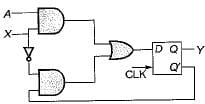The flip-flops are positive edge triggered DFFs. Each state is designated as a two bit string Q0Q1 Let the initial state be 00. The state transition sequence is:

Solution: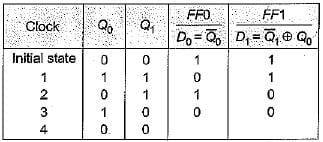The state transitions sequence is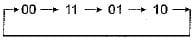QUESTION: 7

The functional difference between SR flip-flop and JK flip-flop is that JK flip-flop

Solution:

S-R flip flop gives invalid output when both inputs are 1 while JK flip-flop toggle the previous output when both inputs are 1. Hence it accepts both inputs 1.

QUESTION: 8

The number of flip-flops required in a modulo N counter is

Solution:

The number of flip-flops required for modulo N counter is [ log2(N)].

QUESTION: 9

Consider the figure given below: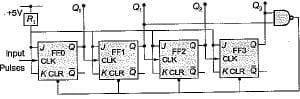​Initially all flip-flops are cleared. How many dock pulse have to be applied to the system before the output from FF3 becomes a HIGH level?

Solution: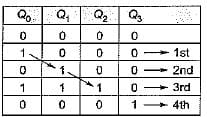So 4 clock pulses are needed.

QUESTION: 10

​The logic circuit shown below is a 3-bit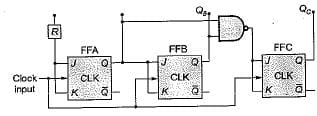Solution:

If input initially are QAQBQC = 000
So after 1st clock pulse QAQBQC = 100
Since, values is incremented so a binary up counter, synchronous since clock provided to all at same time.

QUESTION: 11

The inputs of the J-K flip-flop, shown below are:
PRESET = CLEAR = 1; J = K = 0
If a single clock pulse is applied the device will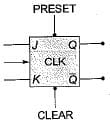Solution:

To make flip-flop working both preset = apart = 1 Since J = K = 0, so it does not change its state i.e. remains in same state.

QUESTION: 12

Consider the following control circuit which contains a 3-bit register and a black box with some combinational logic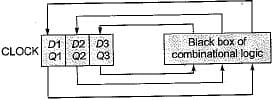The initial state of the circuit is Q1 Q2 Q3 = 000.
The circuit generates the control sequence.
(010) → ( 110) → ( 001) →(001) → . . . → ( 001)
On successive clock cycles. Which of the following sets of equations are implemented by the combinational logic in the black box?

Solution: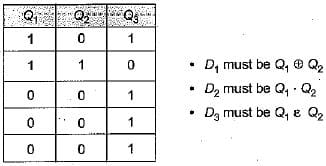QUESTION: 13

‘n’ flip-flop will divide the clock frequency by a factor of

Solution:

n-fiip-flop divide the clock frequency by a factor of 2n.

QUESTION: 14

Let an an-1 ... a1 a0 be the binary representation of an integer b. The integer b is divisible by 3 if

Solution:

Binary representation of 12 is 110 D
So, (q0 + q2)-{q1 + q3)
= (0 + 1)- ( 0 + 1)
= 1 - 1
= 0
0 is divisible by 3 so, true.

QUESTION: 15

If a clock with time period 'T' is used with n stage shift register, the output of final stage will be delayed by

Solution:

N stage shift register will take (n - 1) x clock time to show the output of final stage.Use Code STAYHOME200 and get INR 200 additional OFF Use Coupon Code